# 最短路径——迪杰斯特拉算法

kcoufee

0收藏

• 确定起点的最短路径问题 -即已知起始结点，求最短路径的问题。
• 确定终点的最短路径问题 -与确定起点的问题相反，该问题是已知终结结点，求最短路径的问题。在无向图中该问题与确定起点的问题完全等同，在有向图中该问题等同于把所有路径方向反转的确定起点的问题。
• 确定起点终点的最短路径问题 -即已知起点和终点，求两结点之间的最短路径。
• 全局最短路径问题 -求图中所有的最短路径。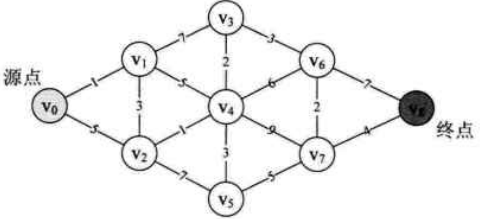1.算法简介

Dijkstra算法是由E.W.Dijkstra于1959年提出，又叫迪杰斯特拉算法，它应用了贪心算法模式，是目前公认的最好的求解最短路径的方法。算法解决的是有向图中单个源点到其他顶点的最短路径问题，其主要特点是每次迭代时选择的下一个顶点是标记点之外距离源点最近的顶点。但由于dijkstra算法主要计算从源点到其他所有点的最短路径，所以算法的效率较低。

2.算法原理

1.首先，引入一个辅助向量D，它的每个分量 D [i]

2.D的初始状态为：若从v 到vi   有弧（即从v 到  vi   存在连接边），则D[i]   为弧上的权值（即为从  v   到vi   的边的权值）；否则置D[i]   为∞。 显然，长度为 D  [j]   = Min{ D |  vj   ∈V } 的路径就是从v   出发到顶点vj   的长度最短的一条路径，此路径为(v,vj   )。

3.那么，下一条长度次短的是哪一条呢？也就是找到从源点v 到下一个顶点的最短路径长度所对应的顶点，且这条最短路径长度仅次于从源点v   到顶点  vj   的最短路径长度。 假设该次短路径的终点是vk ，则可想而知，这条路径要么是(  v,vk   )，或者是(v,vj,vk   )。它的长度或者是从v 到vk   的弧上的权值，或者是D  [j]   加上从vj   到vk   的弧上的权值。

4.一般情况下，假设S为已求得的从源点v 出发的最短路径长度的顶点的集合，则可证明：下一条次最短路径（设其终点为x ）要么是弧(v,x   )，或者是从源点       出发的中间只经过S中的顶点而最后到达顶点       的路径。 因此，下一条长度次短的的最短路径长度必是D[j] = Min{ D[i]   |vi   ∈V-S }，其中D  [i]   要么是弧(  v,vi   )上的权值，或者是D  [k]   (  vk   ∈S)和弧(  vk   ,  vi   )上的权值之和。

3、算法实例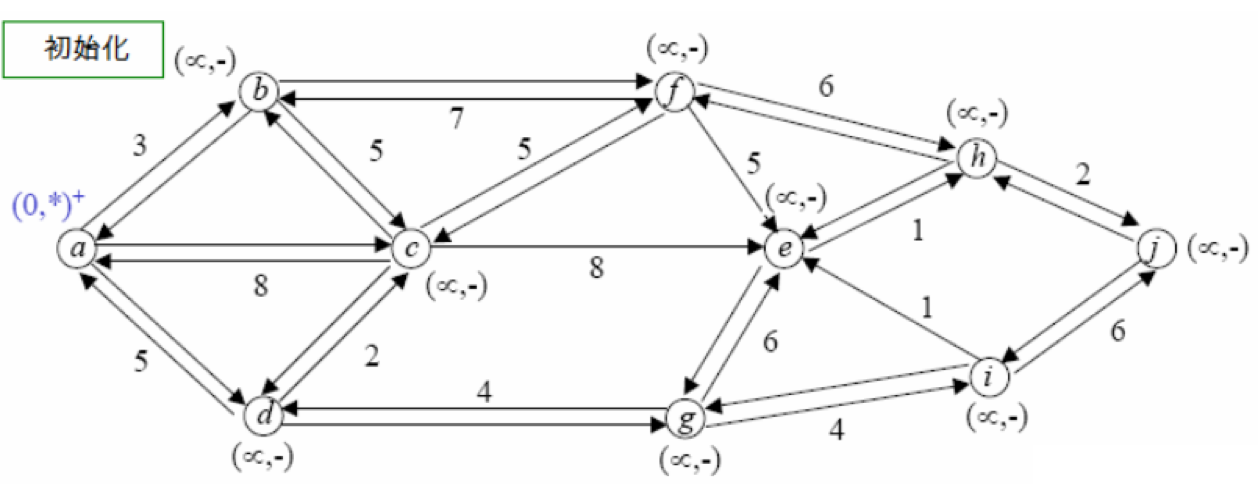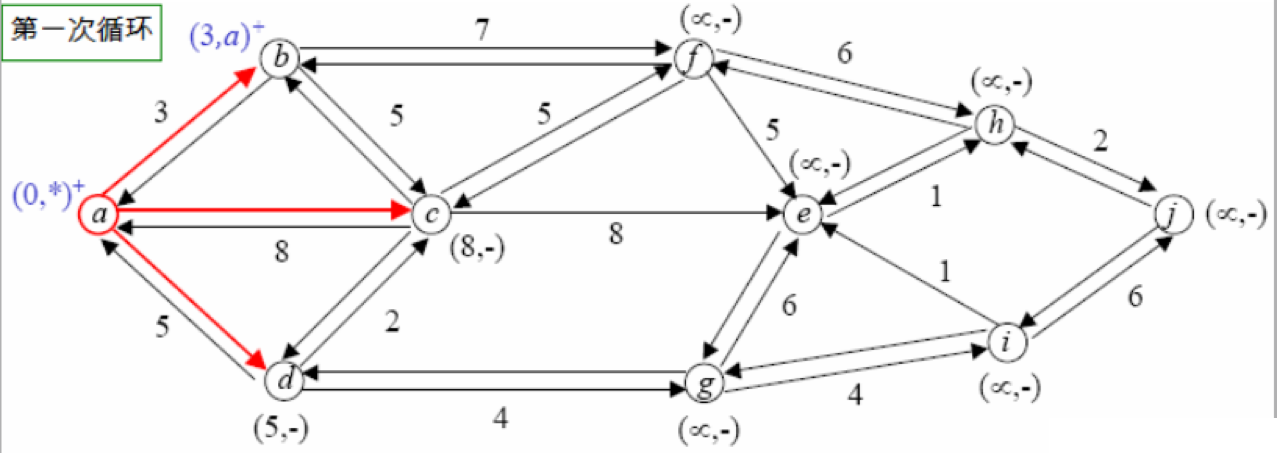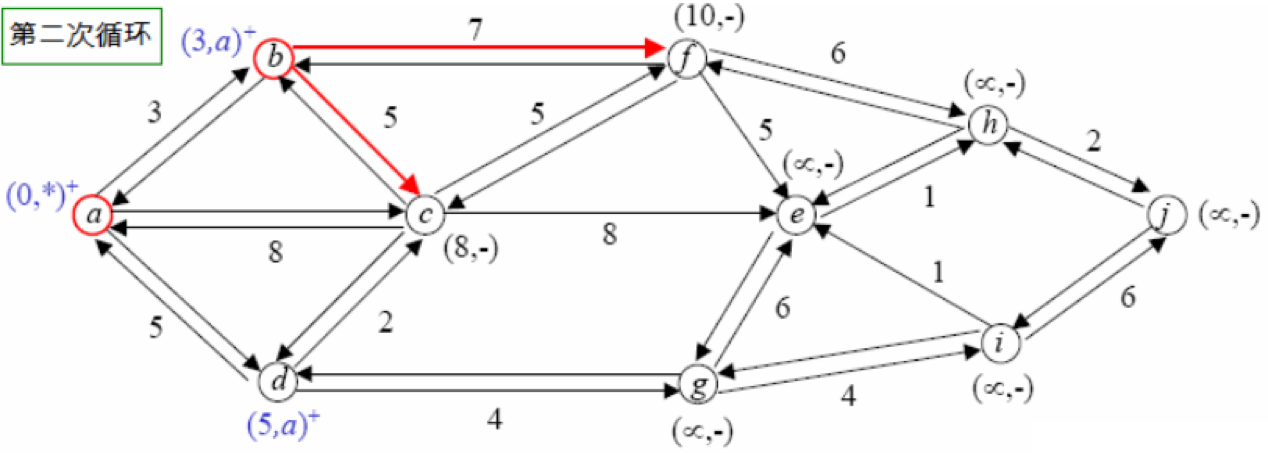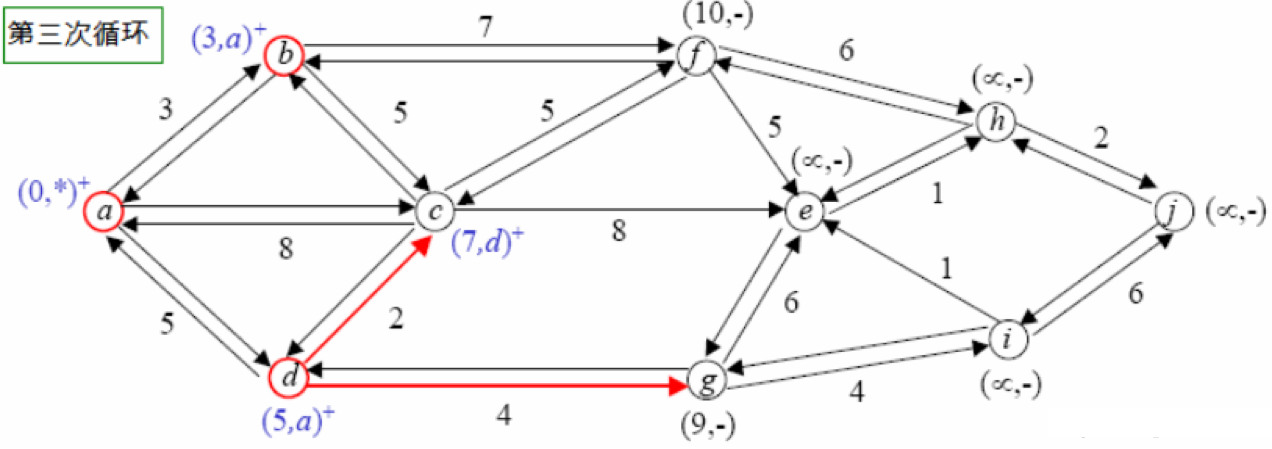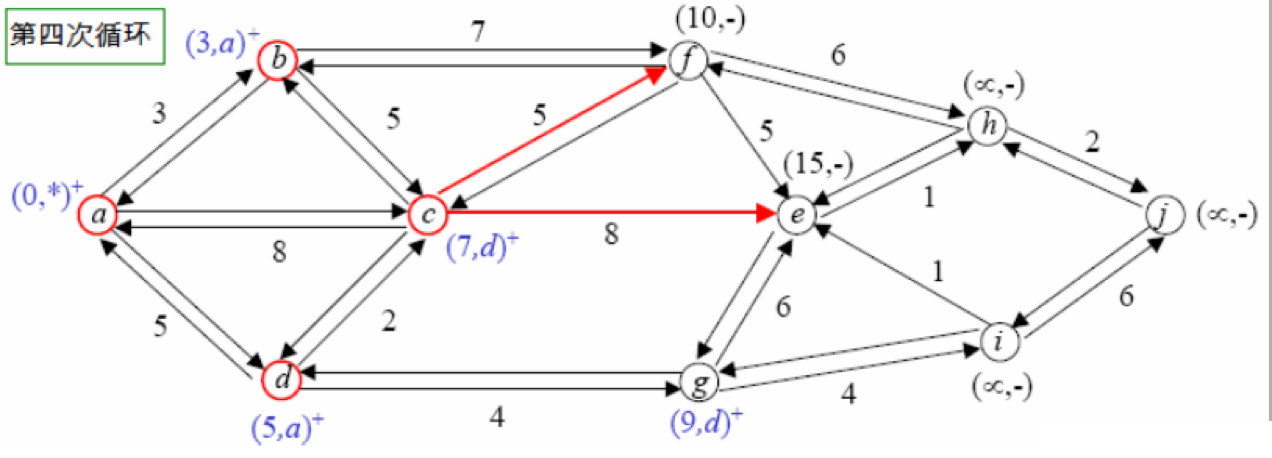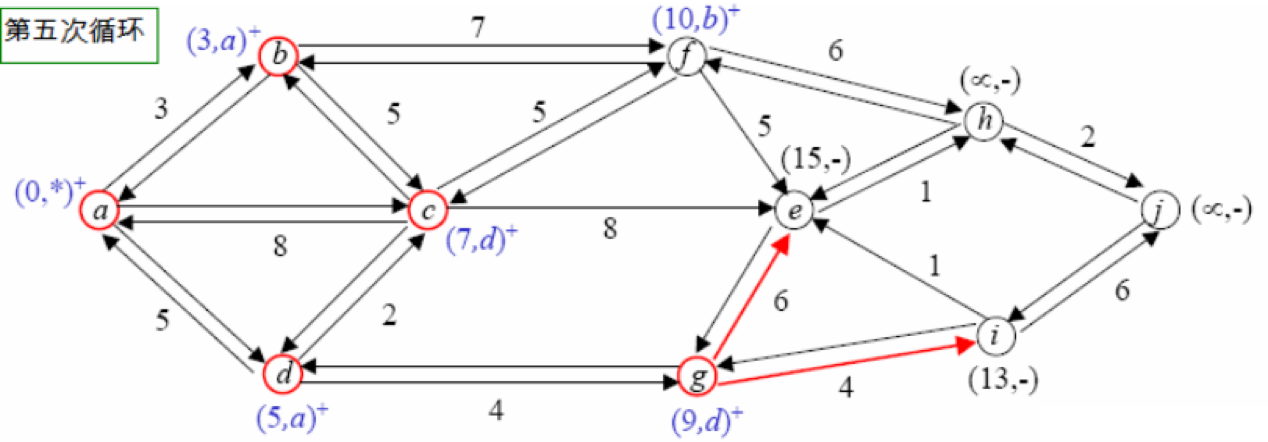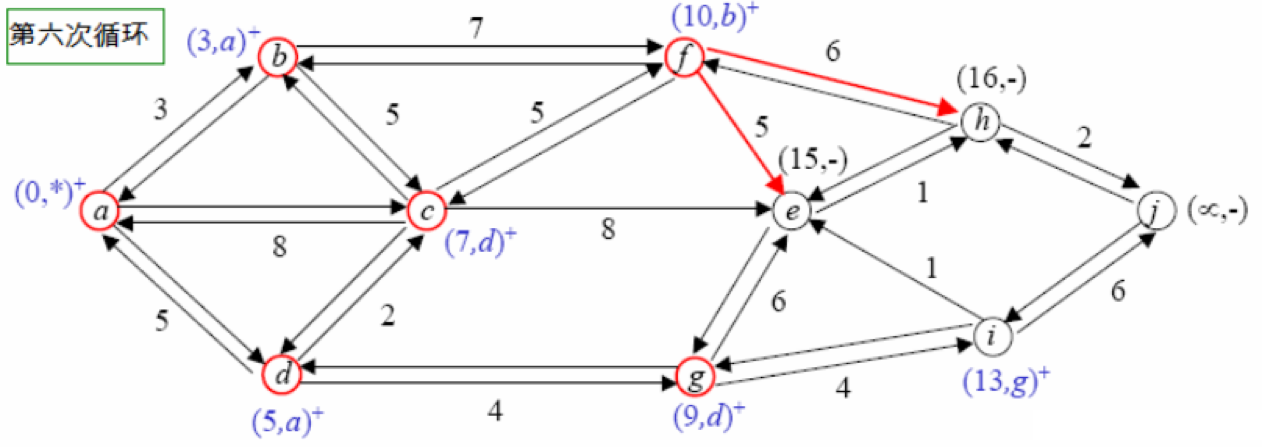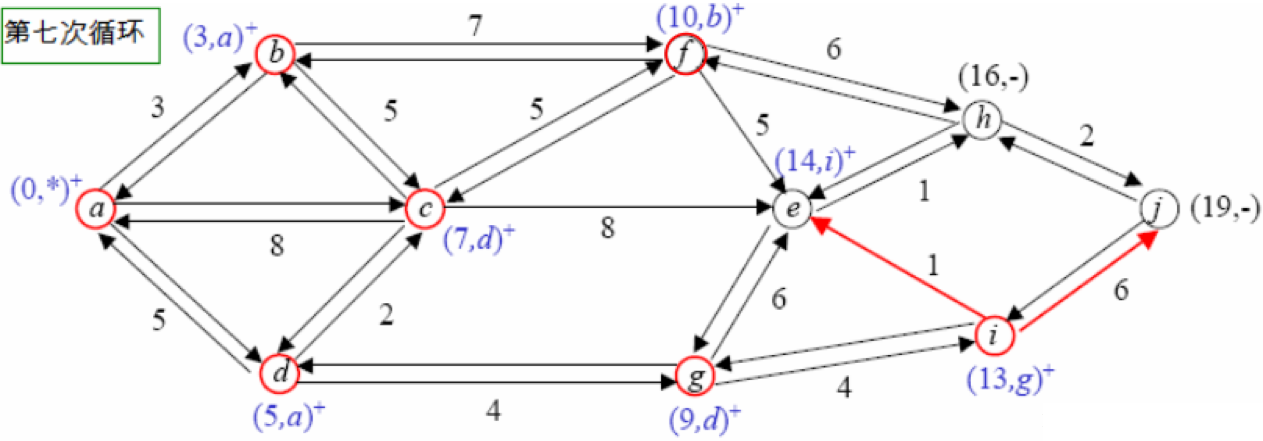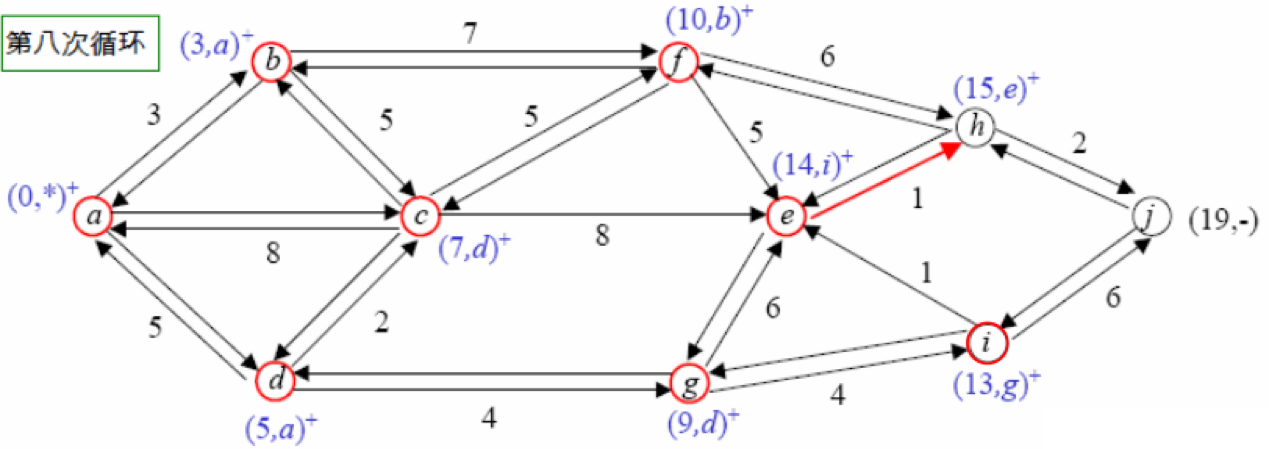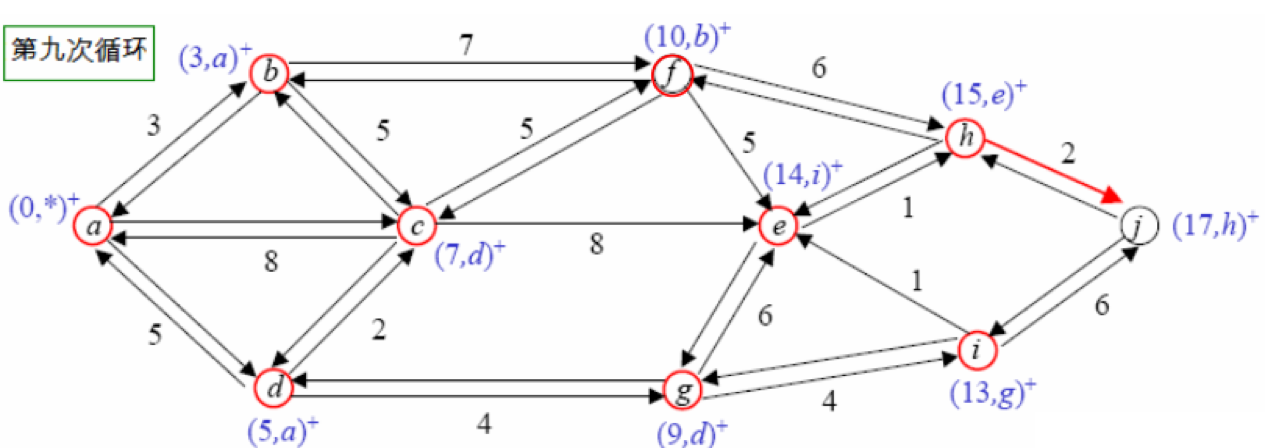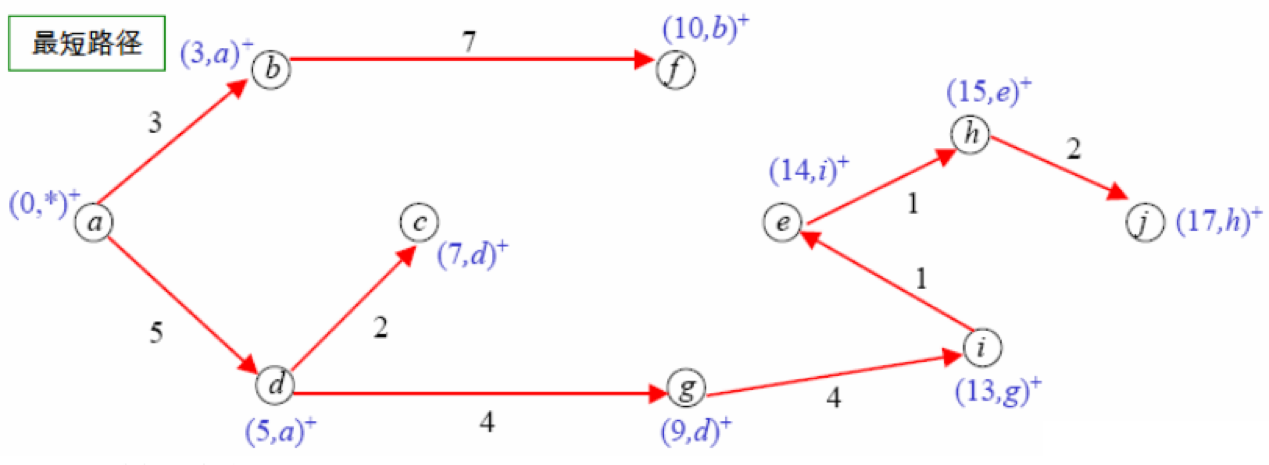4、算法实现

``````#include<stdio.h>
#include<stdlib.h>
#define max 11000000000
inta;
intd;//d表示某特定边距离
intp;//p表示永久边距离
inti,j,k;
intm;//m代表边数
intn;//n代表点数
intmain()
{
scanf("%d%d",&n,&m);
intmin1;
intx,y,z;
for(i=1;i<=m;i++)
{
scanf("%d%d%d",&x,&y,&z);
a[x][y]=z;
a[y][x]=z;
}
for(i=1;i<=n;i++)
d[i]=max1;
d=0;
for(i=1;i<=n;i++)
{
min1=max1;
for(j=1;j<=n;j++)
if(!p[j]&&d[j]<min1)
{
min1=d[j];
k=j;
}
p[k]=j;
for(j=1;j<=n;j++)
if(a[k][j]!=0&&!p[j]&&d[j]>d[k]+a[k][j])
d[j]=d[k]+a[k][j];
}
for(i=1;i<n;i++)
printf("%d->",p[i]);
printf("%d\n",p[n]);
return0;
}``````

5、性能分析帖子
视频
声望
粉丝
热门推荐
社区精华内容